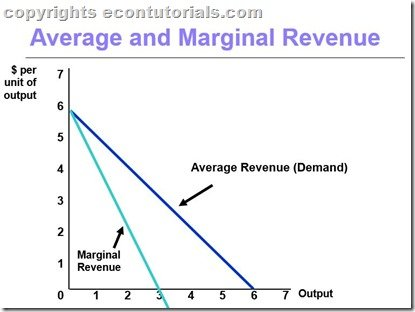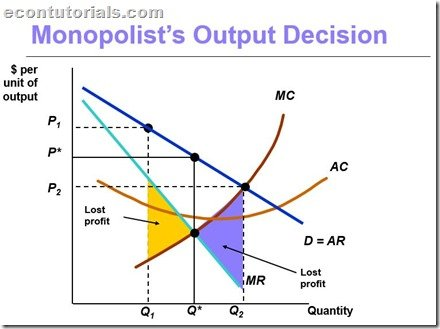Select Page

When firms have some control over the price of their product, we call it an IMPERFECT COMPETITION. This also means that the firm has MARKET POWER; ability to raise price without losing all of the quantity demanded for their product.

Thus,

Market Power + Imperfect Competition = Major sources of Inefficiency

So when we are talking about PURE MONOPOLY, we must keep in account of the following:

1. A monopoly is a Price maker compared to Perfect competition which was a price taker.
2. Single firm producing a product that has no close substitutes
3. Barrier to Entry (Preventing other firms to enter for profit)

So imperfectly competitive firms having a market power must not only decide

1. How much to Produce
2. How to produce it
3. How much to demand in each input market

But also WHAT PRICE TO CHARGE FOR THEIR OUTPUT ?

## Price and Output Decision in Pure Monopoly Markets

To analyze monopoly’s behavior, we make two assumption

1.Entry of new firms is blocked

2. Firms acts in a way that its sole purpose is to maximize profit

It must also be mentioned that a monopolist buys its factors of production just like any other competitive firms. so the labor it hires or the service it requires for the sole production of that product. Additionally, with regard to the cost side of the firm, every monopolist tends to reduce its cost which does not differs from other competitive markets. The difference comes at the point when we are analyzing the demand side which i am going to discuss below.

### Demand in Monopoly Markets

A monopoly market , having one firm shows no distinction between the firm and the industry. So a Monopoly firm is the industry because it is the sole producer. So the demand curve for the market is also the demand curve faced by a firm. And whatever the firm decides to produce is the Quantity supplied of the market.

We nee dot add few more assumptions to make it more simple to understand the whole process. First we need to assume that monopolist does not charges different prices to different customers i.e; price discrimination. It sells its product to all demanders at the same price. We also assume that firm faces a downward demand curve. And by knowing the demand curve it faces, the firm simultaneously chooses both the quantity of output and the price of that output. So when the firm chooses the price, the market determines how much to be sold. This also brings us to the point that monopoly chooses the point on the market demand curve where it wants to be.

### Marginal Revenue and Market Demand

Like any other competitor, a monopolist will choose to produce as long as Marginal revenue exceeds marginal cost. And because the market demand curve is also the demand curve of the monopoly, it is downward sloping.

In Monopoly, increasing the production bring a reduction in the price of its output that it sells. Thus, the revenue that it receives by selling every additional product i.e Marginal Revenue, DECREASES. This analyses also proves why is Marginal Revenue Curve lower than the Monopolist Demand Curve. A monopolist Marginal Revenue Curve Shows the change in total revenue (decreasing change) that results due to increasing output which consequently lowers the price.### Relationship of Average revenue and marginal revenue in monopoly

Average Revenue is the price per unit sold and is precisely the market demand curve of monopoly. Concerning the relationship of Average Revenue and marginal Revenue, we must keep in account of the following facts

1. When Marginal Revenue Increase , so does the Average Revenue and vice versa
2. At a higher price, Nothing is Sold.
3. Because a monopolist wants to increase its sales and therefore increases its production, The Marginal Revenue increases at the beginning of the increase in quantity but eventually reaches negative at a particular point.
4. Note that to increase Sales, Price must fall

## What Quantity should Monopolist Produce ?

We know that Profit maximization point is MR=MC. Lets graphically analyze how a monopoly decides to choose its price and quantity.According to the above graph, Q* is derived from MR=MC intersection that corresponds to Q*. And P* is corresponded from demand curve. Now there are 2 cases incase if the monopolist does not produces at Q*:

1. If monopolist produces at a smaller Quantity , It receives a higher price but at this point the MR>MC.
• In this case, if firm produces a little more (Q1), it would have more revenue-thereby, increasing its profits. And when it keeps on producing , it will eventually reach to the point of Q* making MR=MC ; a point where profits for producing one more unit is Zero. Check the amount of Profit that a monopolist loses which is below the MR curve and above the MC curve.
2. In a second case, If the firm produces more at Q2, MC>MR which means that profits can be increase by reducing Production until it reaches to the point Q*. As this case shows that the cost of Production is more than the revenue it receives from it. So reducing production would be a better option. Thus both the cases are not Preferable for the monopolist.

Thus, both the cases are not recommended for a Monopolist.

### Mathematically

π(Q)=R(Q)-C(Q)

Δπ/ΔQ=ΔR/ΔQ-ΔC/ΔQ=0

MR-MC=0

MR=MC

Shares